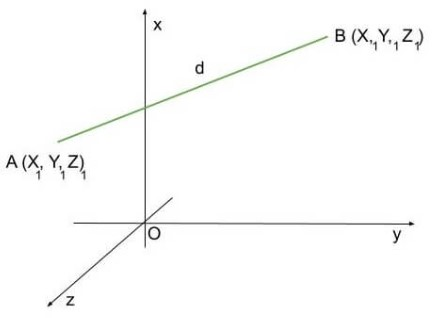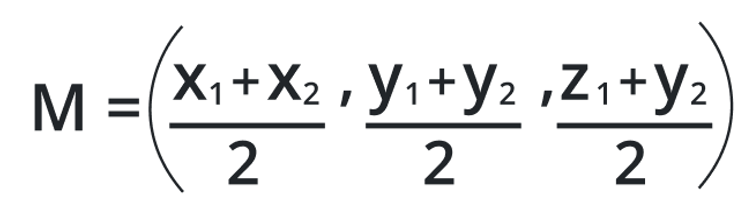# Midpoint (3 Dimensions) Calculator

Enter the 1st and 2nd Coordinates of x, y, and z in the 3d midpoint calculator to calculate midpoints.

Give Us Feedback

## 3d Midpoint Calculator

3d midpoint calculator is a free online tool that finds the average midpoints between different coordinates of a 3d figure. The first answer is the midpoint of x1 and x2 and so on.

## What is the Midpoint?

A midpoint refers to a point in the middle of a line. In 3d shapes, these lines are the ones joining the coordinatesIn a straight line, there is one midpoint. In a 2D shape, there are 2 midpoints, and so on.

## Midpoint Formula

The formulas for finding x, y, and z coordinates are:### For X:

`(X1 + X2) / 2`

### For Y:

`(Y1 + Y2) / 2`

### For Z:

`(Z1 + Z2)  / 2`

## How to find the midpoint?

Example:

Find the midpoints of the coordinates:

X1, Y1, Z1 =  8, 4, 6

X2, Y2, Z2 =  6, 6, 8

Solution:

For X:

= (X1 + X2) / 2

= (8 + 6) / 2

`= 7`

For Y:

= (Y1  +  Y2) / 2

= (4 + 6)  / 2

`= 5`

For Z:

= (Z1 + Z2) / 2

= (6 + 8) / 2

`= 7`

` Midpoints = (7, 5, 7)`

### Math Tools